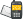It is currently 27 Oct 2020, 04:42### GMAT Club Daily Prep

#### Thank you for using the timer - this advanced tool can estimate your performance and suggest more practice questions. We have subscribed you to Daily Prep Questions via email.

Customized
for You

we will pick new questions that match your level based on your Timer History

Track

every week, we’ll send you an estimated GMAT score based on your performance

Practice
Pays

we will pick new questions that match your level based on your Timer History

#### Not interested in getting valuable practice questions and articles delivered to your email? No problem, unsubscribe here.# 1/x^3,1/x^2,1/x,x,x^2,x^3Question banks Downloads My Bookmarks Reviews Important topics
Author Message
TAGS:
FounderJoined: 18 Apr 2015
Posts: 13693
GRE 1: Q160 V160Followers: 305

Kudos [?]: 3543 , given: 12654

Expert's post00:00

Question Stats:37% (02:34) correct62% (01:22) wrongbased on 27 sessions
$$\frac{1}{x^3},\frac{1}{x^2},\frac{1}{x},x,x^2,x^3$$

If $$-1<x<0$$ , what is the median for the six numbers in the list above ?

A. $$\frac{1}{x}$$

B. $$x^2$$

C. $$\frac{x^2(x+1)}{2}$$

D. $$\frac{x(x^2+1)}{2}$$

E. $$\frac{x^2+1}{2x}$$
[Reveal] Spoiler: OA

_________________

- Do you want all the GRE Prep Club resources at a glance (sort of ) ?? Look at GRE Prep Club Official LinkTree page
- Do you want all the GRE Prep Club FREE resources in one spot ?? Look at Free GRE Materials - Where to get it!! (2020)
- Do you want all the FREE GRE tests on earth ?? Look at FREE GRE Practice Tests [Collection] - New Edition (2021)
- Do you want to know the GRE score in the Top 25 BS in 7 continents ?? Look at here The GRE average score at Top 25 Business Schools 2020 Ed.
- Need Practice 20 Free GRE Quant Tests available for free with 20 Kudos
- GRE Prep Club Members of the Month: Each member of the month will get three months free access of GRE Prep Club tests.

FounderJoined: 18 Apr 2015
Posts: 13693
GRE 1: Q160 V160Followers: 305

Kudos [?]: 3543 , given: 12654

Expert's post
Post A Detailed Correct Solution For The Above Questions And Get A Kudos.
Question From Our New Project: GRE Quant Challenge Questions Daily - NEW EDITION!

For more theory and practice see our GRE - Math Book
_________________

- Do you want all the GRE Prep Club resources at a glance (sort of ) ?? Look at GRE Prep Club Official LinkTree page
- Do you want all the GRE Prep Club FREE resources in one spot ?? Look at Free GRE Materials - Where to get it!! (2020)
- Do you want all the FREE GRE tests on earth ?? Look at FREE GRE Practice Tests [Collection] - New Edition (2021)
- Do you want to know the GRE score in the Top 25 BS in 7 continents ?? Look at here The GRE average score at Top 25 Business Schools 2020 Ed.
- Need Practice 20 Free GRE Quant Tests available for free with 20 Kudos
- GRE Prep Club Members of the Month: Each member of the month will get three months free access of GRE Prep Club tests.ModeratorJoined: 09 Jun 2020
Posts: 164
Followers: 2

Kudos [?]: 122  , given: 9

1
KUDOS
let x value be -0.1

given set will be -1000,100,-10,-0.1,0.01,-0.001

writing them in ascending order -1000,-10,-0.1,-0.001,0.01,100

median will be (-0.1-0.001)/2 =-0.0505

lets verify options
A) (1/x)=-10 not correct

B) $$x^2$$=0.01not correct

C) $$x^2(x+1)/2$$=0.0045not correct

D)$$x(x^2 +1)/2$$=-0.0505correct

E)$$(x^2+1)/2x$$=5.05 not correct

so option D is correct
_________________

thank you
gajala

"If you like my explanation please click kudos"
Thank you for 100 kudos

for more quant problems here is the link

https://greprepclub.com/forum/gre-math- ... -2609.htmlManagerJoined: 08 Aug 2020
Posts: 86
Followers: 1

Kudos [?]: 73  , given: 7

1
KUDOS
From the given condition we can pick our x = -1/2
Then the set become 4,1/4, -1/2,-1/8,-2,-8
Our median is x+x^3/2 =x(x^2+1)/2
Hence D

Posted from my mobile deviceInternJoined: 03 Oct 2020
Posts: 2
Followers: 0

Kudos [?]: 2  , given: 1

2
KUDOS
Given the inequality -1 <x< 0, the value of x has to be a negative fraction.

Therefore, the values 1/(x^2) and x^2 have to be the greatest values as they will result in positive values.

The values 1/x and 1/x^3 have to be the smallest values as they will result in negative integer values.
Say, the value of x = -1/3, then 1/x will be -3 and the value for 1/x^3 will be (-3)^3. Giving the value as integer values.

The two middle values of this list will therefore be x^3 and x. Dividing these two values by x to get the median will be-
x^3+x/2 which gives x(x^2+1)/2

This gives us the answer D

The most conclusive way to do it would be to take a value for x, for instance -1/3 and plug in the values to get the median.
InternJoined: 26 Jan 2020
Posts: 12
Followers: 0

Kudos [?]: 8 , given: 1

for -1<x<0
x^2>x
x^3>x
=> x^2>x^3>x
1/x^2 > x^2
1/x^3 is the least value
and 1/x > 1/x^3

Finally, 1/x^2> x^2 > x^3> x > 1/x > 1/x^2

notice that the middle terms are: x^3 and x

=> median = x^3+x/2
pick DRe: 1/x^3,1/x^2,1/x,x,x^2,x^3   [#permalink] 27 Oct 2020, 01:52
Display posts from previous: Sort by

# 1/x^3,1/x^2,1/x,x,x^2,x^3Question banks Downloads My Bookmarks Reviews Important topicsPowered by phpBB © phpBB Group Kindly note that the GRE® test is a registered trademark of the Educational Testing Service®, and this site has neither been reviewed nor endorsed by ETS®.